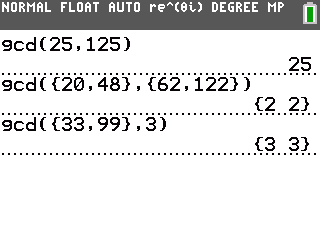# Knowledge Base

## Solution 34793: Calculating the GCD() (Greatest Common Divisor) on the TI-83 Plus and TI-84 Plus Family of Graphing Calculators.

### How do I calculate the gcd() (greatest common divisor) on the TI-83 Plus and TI-84 Plus family of graphing calculators?

The gcd() function is used to calculate the greatest common divisor of two arguments of which must be nonnegative integers. When listA and listB are specified, gcd( returns a list of the gcd of each pair of elements. If list and value are specified, gcd( finds the gcd of each list and value. The proper syntax for the gcd() functions are listed below.

gcd(valueA,valueB)

gcd({listA},{listB})

gcd({list}, value)

The gcd() function can be located by following the keystrokes provided below.

To find gcd of two values:

• Press [MATH] [►] .
• Press   [,].
• Press   .
• Press [)] [ENTER].

To find the gcd of two lists:

• Press [MATH] [►] .
• Press [{]   [,].
• Press   [}].
• Press [,] [{]   [,].
• Press    [}].
• Press [)] [ENTER].

To find the gcd between a list and a value:

• Press [MATH] [►] .
• Press [{]   [,].
• Press   [}].
• Press [,]  [)].
• Press [ENTER].Please see the TI-83 Plus and TI-84 Plus Family guidebooks for additional information.

Last updated: 6/15/2023